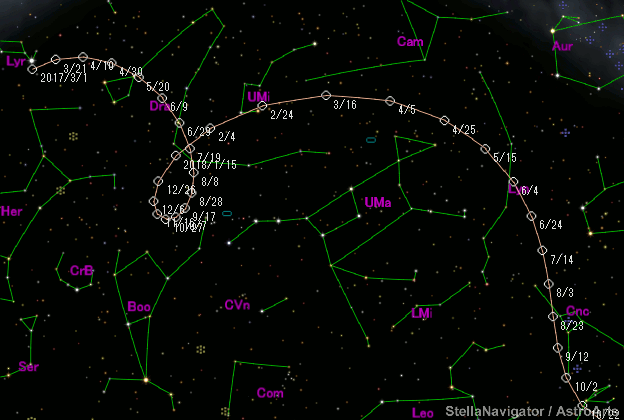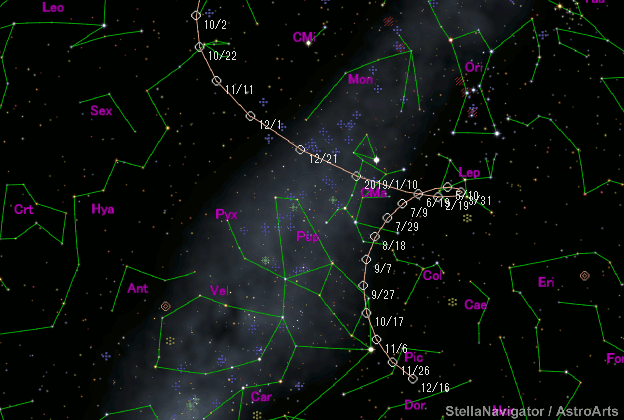# \$B%Q%s%9%?!<%:WB@1(B

C/2016 N6 ( PanSTARRS )###\$B%W%m%U%#!<%k(B

 \$BH/8+F|(B 2016\$BG/(B7\$B7n(B14\$BF|(B \$BH/8+8wEY(B 20.7\$BEy(B \$BH/8+ Pan-STARRS 1 telescope (Haleakala)

###\$B###\$B50F;MWAG(B

```Epoch 2018 July 21.0 TT = JDT 2458320.5
T 2018 July 18.21067 TT                                 Rudenko
q   2.6690703            (2000.0)            P               Q
z  +0.0004677      Peri.  162.80587     -0.53335016     +0.08479677
+/-0.0000001      Node   298.97626     +0.61778889     +0.71869321
e   0.9987518      Incl.  105.83230     +0.57781874     -0.69013737
From 3023 observations 2016 June 27-2020 Feb. 29, mean residual 0".4.
```

###\$B@1?^(B###\$B8wEYJQ2=(B

```        m1 = 3.5 + 5 log\$B&\$(B + 14.0 log r  [ ,0]  (              \$B!A(B2018\$BG/(B 7\$B7n(B18\$BF|(B)
m1 = 4.3 + 5 log\$B&\$(B + 12.0 log r  [0, ]  (2018\$BG/(B 7\$B7n(B18\$BF|!A(B              )
```##### \$B50F;MWAG\$O(BM.P.E.C. 2020-G65\$B\$K7G:\\$5\$l\$?\$b\$N\$G\$9!#(B \$B@1?^\$O%9%F%i%J%S%2!<%?(B Ver.10 (\$B%"%9%H%m%"!<%D(B) \$B\$G:n@.\$7\$?\$b\$N\$G\$9!#(B \$B8wEY%0%i%U\$O(BComet for Windows\$B\$G:n@.\$7\$?\$b\$N\$G\$9!#(B